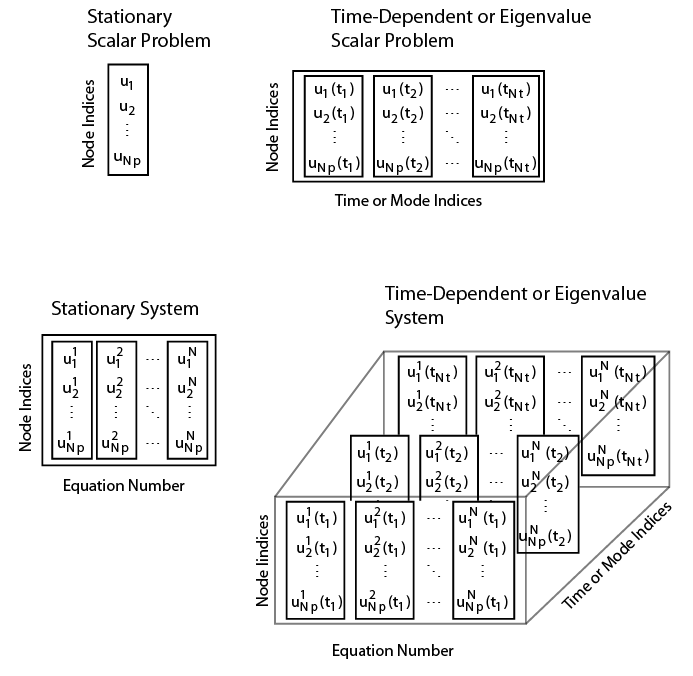## Dimensions of Solutions, Gradients, and Fluxes

`solvepde` returns a `StationaryResults` or `TimeDependentResults` object whose properties contain the solution and its gradient at the mesh nodes. You can interpolate the solution and its gradient to other points in the geometry by using `interpolateSolution` and `evaluateGradient`. You also can compute flux of the solution at the mesh nodes and at arbitrary points by using `evaluateCGradient`.

Note

`solvepde` does not compute components of flux of a PDE solution. To compute flux of the solution at the mesh nodes, use `evaluateCGradient`.

`solvepdeeig` returns an `EigenResults` object whose properties contain the solution eigenvectors calculated at the mesh nodes. You can interpolate the solution to other points by using `interpolateSolution`.

The dimensions of the solution, its gradient, and flux of the solution depend on:

• The number of geometric evaluation points.

• For results returned by `solvepde` or `solvepdeeig`, this is the number of mesh nodes.

• For results returned by `interpolateSolution`,`evaluateGradient`, and `evaluateCGradient` this is the number of query points.

• The number of equations.

• For results returned by `solvepde` or `solvepdeeig`, this is the number of equations in the system.

• For results returned by `interpolateSolution`,`evaluateGradient`, and `evaluateCGradient`, this is the number of query equation indices.

• The number of times for a time-dependent problem or number of modes for an eigenvalue problem.

• For results returned by `solvepde`, this is the number of solution times (specified as an input to `solvepde`).

• For results returned by `solvepdeeig`, this is the number of eigenvalues.

• For results returned by `interpolateSolution`, `evaluateGradient`, and `evaluateCGradient`, this is the number of query times for time-dependent problems or query modes for eigenvalue problems.Suppose you have a problem in which:

• `Np` is the number of nodes in the mesh.

• `Nt` is the number of times for a time-dependent problem or number of modes for an eigenvalue problem.

• `N` is the number of equations in the system.

Suppose you also compute the solution, its gradient, or flux of the solution at other points ("query points") in the geometry by using `interpolateSolution`, `evaluateGradient`, or `evaluateCGradient`, respectively. Here:

• `Nqp` is the number of query points.

• `Nqt` is the number of query times for a time-dependent problem or number of query modes for an eigenvalue problem.

• `Nq` is the number of query equations indices.

The tables show how to index into the solution returned by `solvepde` or `solvepdeeig`, where:

• `iP` contains the indices of nodes.

• `iT` contains the indices of times for a time-dependent problem or mode numbers for an eigenvalue problem.

• `iN` contains the indices of equations.

The tables also show the dimensions of solutions, gradients, and flux of the solution at nodal locations (returned by `solvepde`,`solvepdeeig`, and `evaluateCGradient`) and the dimensions of interpolated solutions and gradients (returned by `interpolateSolution`, `evaluateGradient`, and `evaluateCGradient`).

Stationary PDE problemAccess solution and components of gradientSize of `NodalSolution`, `XGradients`, `YGradients`, `ZGradients`, and components of flux at nodal pointsSize of solution, components of gradient, and components of flux at query points
Scalar

`result.NodalSolution(iP)`

`result.XGradients(iP)`

`result.YGradients(iP)`

`result.ZGradients(iP)`

`Np`-by-1`Nqp`-by-1
System, `N` > `1`

`result.NodalSolution(iP,iN)`

`result.XGradients(iP,iN)`

`result.YGradients(iP,iN)`

`result.ZGradients(iP,iN)`

`Np`-by-`N``Nqp`-by-`N`
Time-dependent PDE problemAccess solution and components of gradientSize of `NodalSolution`, `XGradients`, `YGradients`, `ZGradients`, and components of flux at nodal pointsSize of solution, components of gradient, and components of flux at query points
Scalar

`result.NodalSolution(iP,iT)`

`result.XGradients(iP,iT)`

`result.YGradients(iP,iT)`

`result.ZGradients(iP,iT)`

`Np`-by-`Nt``Nqp`-by-`Nqt`
System, `N` > `1`

`result.NodalSolution(iP,iN,iT)`

`result.XGradients(iP,iN,iT)`

`result.YGradients(iP,iN,iT)`

`result.ZGradients(iP,iN,iT)`

`Np`-by-`N`-by-`Nt``Nqp`-by-`Nq`-by-`Nqt`
PDE eigenvalue problemAccess eigenvectorsSize of `Eigenvectors`Size of interpolated eigenvectors
Scalar

`result.Eigenvectors(iP,iT)`

`Np`-by-`Nt``Nqp`-by-`Nqt`
System, `N` > `1`

`result.Eigenvectors(iP,iN,iT)`

`Np`-by-`N`-by-`Nt``Nqp`-by-`Nq`-by-`Nqt`